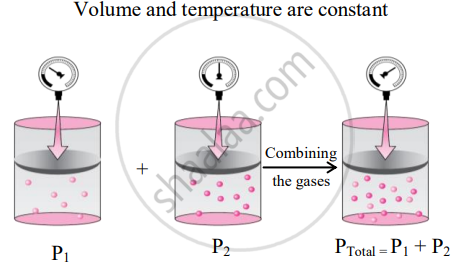# State and write the mathematical expression for Dalton’s law of partial pressure and explain it with a suitable example. - Chemistry

State and write the mathematical expression for Dalton’s law of partial pressure and explain it with a suitable example.

#### Solution

Dalton’s law of partial pressure:

1. Statement: The total pressure of a mixture of two or more non-reactive gases is the sum of the partial pressures of the individual gases in the mixture.
2. Explanation:
Dalton’s law can be mathematically expressed as:
PTotal = P1 + P2 + P3 …(at constant T and V)
where, PTotal is the total pressure of the mixture and P1, P2, P3, … are the partial pressures of individual gases 1, 2, 3, … in the mixture.
For example, consider two non-reactive gases A and B. On mixing the two gases, the pressure exerted by individual gas A in the mixture of both the gases is called partial pressure of gas A (say P1). Likewise, the partial pressure of gas B is P2. According to Dalton’s law, the total pressure of the mixture of gas A and B at constant T and V will be given as:
PTotal = P1 + P2
3. Schematic illustration of Dalton’s law of partial pressures:Concept: Ideal Gas Equation
Is there an error in this question or solution?
Chapter 10: States of Matter - Exercises [Page 158]

#### APPEARS IN

Balbharati Chemistry 11th Standard Maharashtra State Board
Chapter 10 States of Matter
Exercises | Q 4. (A) | Page 158
Share